# [Solved] When multiplying both sides of an equation does not lead to a solution

#### dcbingaman

Joined Jun 30, 2021
691
Consider the following equation: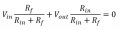This can be solved for Vout/Vin in the following way: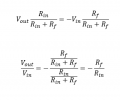But what happens when I take the original equation and multiply by Rin+Rf on both sides to eliminate the denominator term as such: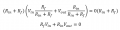I know intuitively that the answer is wrong and that you cannot simply multiply an equation of the form x/y+z/y=0 by y. How do you know when you can multiply both sides by a constant and when you cannot? It seems multiplying by a constant when one side of the equation equals zero is not allowed.

#### Alec_t

Joined Sep 17, 2013
13,077
RfVin+RinVout=0
Rf+RinVout/Vin=0
Vout/Vin+Rf/Rin=0
Vout/Vin= -Rf/Rin

•dcbingaman

#### dcbingaman

Joined Jun 30, 2021
691
Naturally! Not sure why I did not see that, guess not enough coffee this morning.

•nsaspook and MrSalts

#### WBahn

Joined Mar 31, 2012
26,932
The issue has nothing to do with one side being zero.

The issue has to do with the constraints implied by the original equation.

Because you have (Rin + Rf) in the denominator, you are implying that (Rin + Rf) does NOT equal zero because, if it does, then both terms go to infinity and you have one voltage times infinity plus another voltage times infinity infinity equals zero, which may or may not have any useful interpretation.

You have this same issue with both methods you used, and both methods yield the same result (as has already been pointed out). Now you just need to ask yourself what it takes for (Rin + Rf) to be zero and whether or not that can even ever happen. If it can't, no need to worry about it. If it can, then you need to worry about it and delve deeper to determine what would happen in that situation.

BTW, asking (and answering) those kinds of questions have lead to revolutionary discoveries, be it new circuits that do really interesting things, or even major discoveries in physics -- not to mention more than one interesting plot line in a sci-fi story.

•panic mode and dcbingaman

#### dcbingaman

Joined Jun 30, 2021
691
The issue has nothing to do with one side being zero.

The issue has to do with the constraints implied by the original equation.

Because you have (Rin + Rf) in the denominator, you are implying that (Rin + Rf) does NOT equal zero because, if it does, then both terms go to infinity and you have one voltage times infinity plus another voltage times infinity infinity equals zero, which may or may not have any useful interpretation.

You have this same issue with both methods you used, and both methods yield the same result (as has already been pointed out). Now you just need to ask yourself what it takes for (Rin + Rf) to be zero and whether or not that can even ever happen. If it can't, no need to worry about it. If it can, then you need to worry about it and delve deeper to determine what would happen in that situation.

BTW, asking (and answering) those kinds of questions have lead to revolutionary discoveries, be it new circuits that do really interesting things, or even major discoveries in physics -- not to mention more than one interesting plot line in a sci-fi story.
Nice points! Last time I checked, it is next to impossible to have zero and or negative resistance values.#### ci139

Joined Jul 11, 2016
1,869
the site supports the $${\large \LaTeX}$$ notation (($$\leftarrow$$ you can see the source by right cliking the equations and selecting Show Math As > TeX Commands))

considering the non-inverting input has an arbitrary potential of $$V_{REF}$$ the following applies :

$${\huge \frac{V_{OUT}-V_{IN}}{R_{IN}+R_{F}}=\frac{V_{REF}-V_{IN}}{R_{IN}}=\frac{V_{OUT}-V_{REF}}{R_{F}}=I_{R_F}=I_{R_{IN}}}$$

so your equation becomes

$${\huge A_V=\frac{V_{OUT}-V_{REF}}{V_{IN}-V_{REF}}=-\frac{R_F}{R_{IN}}}$$

where the need for multiplication kind of drops off

•dcbingaman

#### WBahn

Joined Mar 31, 2012
26,932
Nice points! Last time I checked, it is next to impossible to have zero and or negative resistance values.You actually can have negative resistances -- particularly in small-signal equivalent circuits or circuits with dependent supplies. So there are instances where the question is relevant. But I'm guessing that you are working with something like a pretty standard op amp circuit with passive component values, and so recognizing that you can't have zero or negative values is useful. What's also useful is to consider how you would expect the circuit to behave in the limit that both Rf and Rin go to zero, taking into account that, as they both get very small, one will still almost always be many times larger than the other, but you won't know which. So, what would you expect to happen? And would some other limiting factor in the circuit come into play at some point? If so, at what point?

•dcbingaman

#### dcbingaman

Joined Jun 30, 2021
691
the site supports the $${\large \LaTeX}$$ notation (($$\leftarrow$$ you can see the source by right cliking the equations and selecting Show Math As > TeX Commands))

considering the non-inverting input has an arbitrary potential of $$V_{REF}$$ the following applies :

$${\huge \frac{V_{OUT}-V_{IN}}{R_{IN}+R_{F}}=\frac{V_{REF}-V_{IN}}{R_{IN}}=\frac{V_{OUT}-V_{REF}}{R_{F}}=I_{R_F}=I_{R_{IN}}}$$

so your equation becomes

$${\huge A_V=\frac{V_{OUT}-V_{REF}}{V_{IN}-V_{REF}}=-\frac{R_F}{R_{IN}}}$$

where the need for multiplication kind of drops off
I have been using MS Word for equations. Looks like Latex is useful. Don't know the syntax but is looks fairly straight forward.

#### dcbingaman

Joined Jun 30, 2021
691
You actually can have negative resistances -- particularly in small-signal equivalent circuits or circuits with dependent supplies. So there are instances where the question is relevant. But I'm guessing that you are working with something like a pretty standard op amp circuit with passive component values, and so recognizing that you can't have zero or negative values is useful. What's also useful is to consider how you would expect the circuit to behave in the limit that both Rf and Rin go to zero, taking into account that, as they both get very small, one will still almost always be many times larger than the other, but you won't know which. So, what would you expect to happen? And would some other limiting factor in the circuit come into play at some point? If so, at what point?
I know there are certain circumstances where the dynamic resistance can be negative for small signals such that: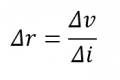Can be negative. 'Dynamic Resistance'.

Never thought about the ideas of taking things close to zero for op-amps. Not practical but sure is an interesting intellectual question. In theory for an ideal op amp, no matter how small the resistance as long as the ratio holds: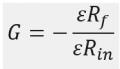Where epsilon is some insanely small rational number the gain is constant.

I am not sure what you are referring to when you state taking each resistance close to zero that one will become significantly closer to zero than the other?

"one will still almost always be many times larger than the other"

I don't see that mathematically, you can make both as small as you want by multiplying both of them by a really small number very close to zero, the ratio remains the same.

The obvious things that would happen in an ideal op-amp circuit:

The input current would increase without limit being the input current is just: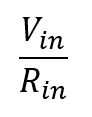The op-amp output current would also increase without limit being it is just Vo/Rf.

The power used would increase without limit! Don't want to pay that electric bill! Going to be sky high! You could also heat your entire house using just this op-amp circuit due to the ridiculous amount of heat given off by the circuit.

You need a power supply with no limits in current.

With a real world op-amp:

The driving of Vin would go towards zero, being no voltage source comes with zero internal resistance.

The op-amp output can only drive so much current, so the output current of the op-amp would approach a constant and stop increasing while the voltage starts to come back down.

So the gain would decrease after a certain point.

You can always go the other way and increase both Rf and Rin without limit. The input impedance would increase without limit, the power used will approach zero. But the op-amp input impedance though high would start to load down the circuit and again the gain would drop. But at least with increasing Rf and Rin you don't end up with an electric bill so high you can never pay it.

Forgot to mention, the electric and magnetic field gradients would be so high as to fry your brain if you are anywhere in the vicinity of this circuit. The inductance and capacitance of the circuit needs to be both practically zero for the current to go up without limit.Last edited:

#### WBahn

Joined Mar 31, 2012
26,932
I am not sure what you are referring to when you state taking each resistance close to zero that one will become significantly closer to zero than the other?

"one will still almost always be many times larger than the other"

I don't see that mathematically, you can make both as small as you want by multiplying both of them by a really small number very close to zero, the ratio remains the same.
On paper, yes, but you need to look beyond an ideal, I-can-control-everything-to-infinite-precision paper world.

Let's say that in trying to reduce Rin and Rf to zero, Rin is reduced from 10 kΩ to 0.18 pΩ and Rf is reduced to 1 kΩ to 0.59 pΩ. Both have been reduced to effectively zero resistance, but the Rf/Rin ratio has gone from 10 to 0.31. As you try to drive a component value to an extreme, particularly a small extreme, other factors that you have little control over start to dominate, such as lead wire resistance, or PCD track routing or thickness, or solder joint resistance.

The obvious things that would happen in an ideal op-amp circuit:

The input current would increase without limit being the input current is just:

View attachment 273604

The op-amp output current would also increase without limit being it is just Vo/Rf.

The power used would increase without limit! Don't want to pay that electric bill! Going to be sky high! You could also heat your entire house using just this op-amp circuit due to the ridiculous amount of heat given off by the circuit.

You need a power supply with no limits in current.

With a real world op-amp:
...
And these are the kinds of things that need to be thought of -- and that a dismaying number of people fail to do (so good on you for not doing so). It's not just students that fall into the trap of thinking that the equations on paper are perfect representations of the real world.

•dcbingaman

#### dcbingaman

Joined Jun 30, 2021
691
On paper, yes, but you need to look beyond an ideal, I-can-control-everything-to-infinite-precision paper world.

Let's say that in trying to reduce Rin and Rf to zero, Rin is reduced from 10 kΩ to 0.18 pΩ and Rf is reduced to 1 kΩ to 0.59 pΩ. Both have been reduced to effectively zero resistance, but the Rf/Rin ratio has gone from 10 to 0.31. As you try to drive a component value to an extreme, particularly a small extreme, other factors that you have little control over start to dominate, such as lead wire resistance, or PCD track routing or thickness, or solder joint resistance.

And these are the kinds of things that need to be thought of -- and that a dismaying number of people fail to do (so good on you for not doing so). It's not just students that fall into the trap of thinking that the equations on paper are perfect representations of the real world.
I think you got the resistor values swapped Rf=10K, Rin=1K for -10 gain.
I think you meant -0.59/0.18 = -3.2 opposed to -10, but I know what you are getting at.
We would run into trouble long before this even 1 ohm to 10 ohm ratio, the poor op-amp could not even drive it. Most normal ones could not.

The thing that makes your example really come to life is the input impedance of 0.18pOhms, for a 100mV input voltage that would be over 500 billion amps at just 0.1V input! The op-amp would have to sink 500 billion amps on the output. This 'resistor' would have to dissipate over 50 billion watts of power! Power in Rf would be even worse.

Last edited:

#### WBahn

Joined Mar 31, 2012
26,932
I think you got the resistor values swapped Rf=10K, Rin=1K for -10 gain.
I think you meant -0.59/0.18 = -3.2 opposed to -10, but I know what you are getting at.
We would run into trouble long before this even 1 ohm to 10 ohm ratio, the poor op-amp could not even drive it. Most normal ones could not.

The thing that makes your example really come to life is the input impedance of 0.18pOhms, for a 100mV input voltage that would be over 500 billion amps at just 0.1V input! The op-amp would have to sink 500 billion amps on the output. This 'resistor' would have to dissipate over 50 billion watts of power! Power in Rf would be even worse.
Yep, I wasn't even looking up at the equations as I typed my off-the-cuff numbers. Should have.

I can't begin to count the number of times I've seen students (and more than a few degreed engineers) take whatever a circuit simulator tells them at face value, including simulations in which a moment's inspection reveals that it is claiming millions of amps being drawn from a supply or some other ridiculous claim.

•dcbingaman

#### MrAl

Joined Jun 17, 2014
9,357
Naturally! Not sure why I did not see that, guess not enough coffee this morning.
Hey dont sweat it ive done worse some late late nights at the drawing board.

The nice thing about having zero on one side is that anything you multiply it by will disappear from the equation. This means that if you have a very complicated denominator on the left and zero on the right, you can effectively get rid of the denominator by multiplying both sides by the denominator. This happens a lot in AC theory.
So for example:
(x+1)/(x^4+x^3+x^2+x+1)=0
multiply by the denominator we get:
x+1=0

and that's a lot simpler.

•dcbingaman

#### dcbingaman

Joined Jun 30, 2021
691
Hey dont sweat it ive done worse some late late nights at the drawing board.

The nice thing about having zero on one side is that anything you multiply it by will disappear from the equation. This means that if you have a very complicated denominator on the left and zero on the right, you can effectively get rid of the denominator by multiplying both sides by the denominator. This happens a lot in AC theory.
So for example:
(x+1)/(x^4+x^3+x^2+x+1)=0
multiply by the denominator we get:
x+1=0

and that's a lot simpler.
That makes a lot of sense after thinking about it. No matter what is in the denominator, there is nothing that I can think of in the denominator that would allow for a rational result that is zero. That is no value of d in the following would result in n/d=0 where n!=0. So n/d=0 where n!=0 has no solutions.

#### WBahn

Joined Mar 31, 2012
26,932
Hey dont sweat it ive done worse some late late nights at the drawing board.

The nice thing about having zero on one side is that anything you multiply it by will disappear from the equation. This means that if you have a very complicated denominator on the left and zero on the right, you can effectively get rid of the denominator by multiplying both sides by the denominator. This happens a lot in AC theory.
So for example:
(x+1)/(x^4+x^3+x^2+x+1)=0
multiply by the denominator we get:
x+1=0

and that's a lot simpler.
In general, this is dangerous. When you get rid of something in the denominator, you need to make note that you now have a different function that may be well-defined at points where the original function wasn't, namely at places where the denominator when to zero. In the particular example, the expression in the denominator never goes to zero for any real value of x, so as long as x can't take on complex values, what you did was safe.

As a simple example, let's change this to

sin(x)/x = 0

Now use your approach and multiply both sides by x, giving

sin(x) = 0

The conclusion would be that since sin(x) = 0 for x = 0, that x = 0 must be one of the solutions of the original problem.

But it's not, because sin(x)/x is undefined at x = 0 and, in the limit that x goes to zero, is 1.

Before multiplying the equation by x to remove it from the denominator, a note needs to be made that the new function equation does not apply to the original equation at x=0 because that is a singularity in the original equation that has been artificially removed in the new one.

•panic mode and dcbingaman

#### MrAl

Joined Jun 17, 2014
9,357
In general, this is dangerous. When you get rid of something in the denominator, you need to make note that you now have a different function that may be well-defined at points where the original function wasn't, namely at places where the denominator when to zero. In the particular example, the expression in the denominator never goes to zero for any real value of x, so as long as x can't take on complex values, what you did was safe.

As a simple example, let's change this to

sin(x)/x = 0

Now use your approach and multiply both sides by x, giving

sin(x) = 0

The conclusion would be that since sin(x) = 0 for x = 0, that x = 0 must be one of the solutions of the original problem.

But it's not, because sin(x)/x is undefined at x = 0 and, in the limit that x goes to zero, is 1.

Before multiplying the equation by x to remove it from the denominator, a note needs to be made that the new function equation does not apply to the original equation at x=0 because that is a singularity in the original equation that has been artificially removed in the new one.
Hi,

Havent seen you around for a while wondered if everything was ok in your neck of the woods.

Yes you are right, when i just calculated:
sin(x)/x=0

and multiplied both sides by x i got:
sin(x)=0

and my whole house blew up It's definitely dangerousYes this is true with many different circumstances whenever we multiply by the variable. This is something that has to be paid attention to. This happens a lot too for example:
x=0
x^2=0
x^3=0
the more we multiply by x the more solutions we get. It's a fact of life that applies in every situation so we have to watch out for that. It's a good point though when it does not seem apparent at first.

It's also interesting that sin(x)=0 has an infinite number of solutions.

There is a catch though, and that is if we state that:
sin(x)/x=0

then limit(sin(x)/x)=1 when x goes to zero doesnt happen to be a solution to this particular problem.
That means we might have to say instead:
sin(x)/x=0 for x not equal to 0.
sin(x)/x=1 for x approaching zero.

•dcbingaman

#### WBahn

Joined Mar 31, 2012
26,932
There is a catch though, and that is if we state that:
sin(x)/x=0

then limit(sin(x)/x)=1 when x goes to zero doesnt happen to be a solution to this particular problem.
And that's the danger I was pointing out with the approach you were recommending to get something out of the denominator when one side is zero. You can't just blindly eliminate the denominator, you must recognize that any place where the denominator goes to zero (or infinity) is a point that needs to be treated specially.

That means we might have to say instead:
sin(x)/x=0 for x not equal to 0.
sin(x)/x=1 for x approaching zero.
This is mixing up two different things. The bottom what is stating what sin(x)/x is equal to as x approaches zero, but the top one, which is worded using the same format, would then be claiming that sin(x)/x is euqal to zero for all x not equal to zero, and that is a false statement.

The original problem was to solve sin(x)/x = 0, which is asking for what values of x is that statement true.

At x = 0, sin(x)/x is indeterminate and has no defined value. This is why the definition of the sinc(x) function needs to specify the value of sinc(0) explicitly and then functionally for all other x.

sin(x)/x = 0

has solutions at x = k*pi for all non-zero integer k.

•dcbingaman

#### MrAl

Joined Jun 17, 2014
9,357
And that's the danger I was pointing out with the approach you were recommending to get something out of the denominator when one side is zero. You can't just blindly eliminate the denominator, you must recognize that any place where the denominator goes to zero (or infinity) is a point that needs to be treated specially.

This is mixing up two different things. The bottom what is stating what sin(x)/x is equal to as x approaches zero, but the top one, which is worded using the same format, would then be claiming that sin(x)/x is euqal to zero for all x not equal to zero, and that is a false statement.

The original problem was to solve sin(x)/x = 0, which is asking for what values of x is that statement true.

At x = 0, sin(x)/x is indeterminate and has no defined value. This is why the definition of the sinc(x) function needs to specify the value of sinc(0) explicitly and then functionally for all other x.

sin(x)/x = 0

has solutions at x = k*pi for all non-zero integer k.
Hello again,

In short, there are a lot of ways of solving these problems and of course you have to watch out for how you solve an equation, but the method does in fact turn up solutions and that was what we were after.
Not only that, it may be the ONLY way to solve some equations. It's a standard practice for solving some equations you probably would not be able to find a solution to some of them without doing this multiplication.